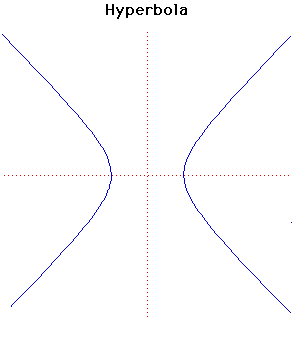# HyperbolaCartesian equation:
x2/a2 - y2/b2 = 1
or parametrically:
x = a sec(t), y = b tan(t)

Click below to see one of the Associated curves.

Click THIS LINK to experiment interactively with this curve and its associated curves.

A special case of the hyperbola was first studied by Menaechmus. This special case was xy = ab where the asymptotes are at right angles and this particular form of the hyperbola is called a rectangular hyperbola.

Euclid and Aristaeus wrote about the general hyperbola but only studied one branch of it while the hyperbola was given its present name by Apollonius who was the first to study the two branches of the hyperbola.

The focus and directrix of a hyperbola were considered by Pappus.

The pedal curve of a hyperbola with its focus as pedal point is a circle. The pedal of a rectangular hyperbola with its centre as pedal point is a lemniscate.

The evolute of the hyperbola with equation given above is the Lamé curve

(ax)2/3 - (by)2/3 = (a + b)2/3.
From a point between the two branches of the evolute two normals can be drawn to the hyperbola but from a point beyond the evolute four normals can be drawn.

If the centre of a rectangular hyperbola is taken as the centre of inversion, the rectangular hyperbola inverts to a lemniscate. If the vertex of a rectangular hyperbola is taken as the centre of inversion, the rectangular hyperbola inverts to a right strophoid. If the focus of a hyperbola is taken as the centre of inversion, the hyperbola inverts to a limacon. In this last case if the asymptotes of the hyperbola make an angle of π/3 with the axis which cuts the hyperbola then it inverts to a Trisectrix of Maclaurin.

Other Web site:

JOC/EFR/BS January 1997